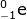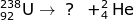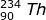# Radioactive Decay Formula

Radioactive Decay Formula

Radioactive decay occurs when an unstable nucleus emits ionizing radiation. The three most common forms of radioactive decay involve alpha, beta, or gamma emission.

In alpha decay (), a particle with a mass number of 4 and atomic number of 2 is emitted from a nucleus. It is the size of a helium atom.

In beta decay (), a particle with a mass number of 0 and atomic number of -1 is emitted from a nucleus. It is referred to as a high speed electron.

In gamma decay (), a particle with no mass or atomic number is emitted from a nucleus. This emission consists of high energy photons.

Radioactive Decay Formula Questions:

1. Identify the particle that forms after the alpha emission of a 238-U atom occurs.Answer:

In order to identify the particle, you must use the mass and atomic numbers of uranium and the alpha particle. The uranium atom has a mass of 238 and loses an alpha particle with a mass of 4. This means the particle formed will have a mass of 234. Likewise, the atomic number of uranium is 92 and loses 2 during alpha emission. The newly formed particle will have a mass of 90.2. Identify the type of emission that occurs in the following radioactive decay.Answer:

Using the mass and atomic numbers, the identity of the radioactive decay can be found. In the case of the mass, both particles (231-Th and 231-Pa) have the same mass. So the emission has a zero mass. The number of protons emitted, however, is not zero. In this case the atomic number of thorium (90) is one less than the atomic number of Pa (91). The mass of the emitted particle must be -1 (90 - -1 = 91). The emitted particle must be a beta particle ().

 Related Links: